# Difference between revisions of "Transport along a curve"

## Definition

Let$M$ be a differential manifold,$E$ a vector bundle on$M$. Let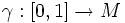$\gamma:[0,1] \to M$ be a smooth curve in$M$. Let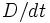$D/dt$ denote a connection along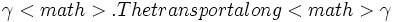$\gamma[itex]. The transport along [itex]\gamma$ defined by$D/dt$ maps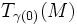$T_{\gamma(0)}(M)$ to the space of vector fields along$\gamma[itex], denoted in symbols as: [itex]v \mapsto \phi_t(v) (t \in [0,1])$

such that for any vector$v \in T_{\gamma(0)}(M)$: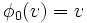$\phi_0(v) = v$

and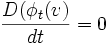$\frac{D (\phi_t(v)}{dt} = 0$

Intuitively, we define a rule for moving the tangent space along the curve, in a manner that is parallel to itself with respect to the connection.